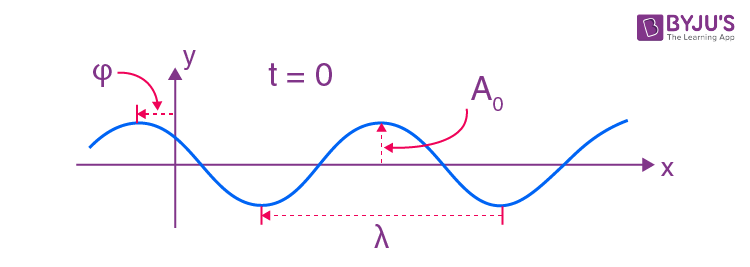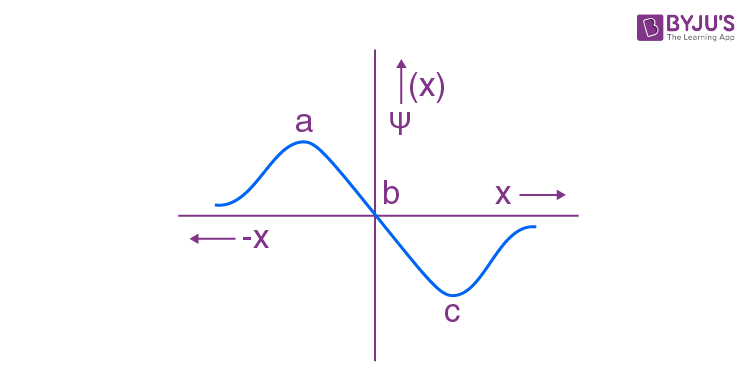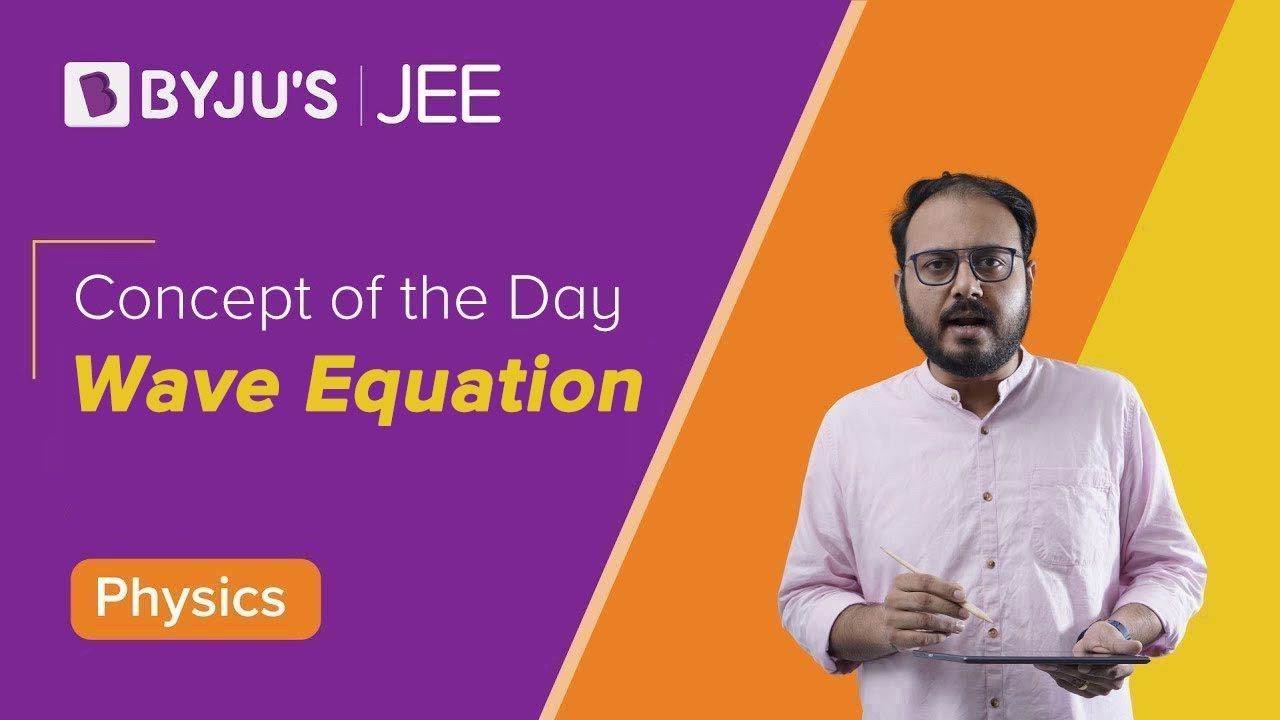Win up to 100% scholarship on Aakash BYJU'S JEE/NEET courses with ABNAT Win up to 100% scholarship on Aakash BYJU'S JEE/NEET courses with ABNAT

# Schrodinger Wave Equation

The Schrodinger wave equation, or just the Schrodinger equation, is one of the most fundamental equations of Quantum Physics and an important topic for the JEE. The equation, also called the Schrodinger equation, is basically a differential equation and is widely used in Chemistry and Physics to solve problems based on the atomic structure of matter.

The Schrodinger wave equation describes the behaviour of a particle in a field of force or the change of a physical quantity over time. Erwin Schrödinger, who developed the equation, was even awarded the Nobel Prize in 1933.

## What Is Schrodinger Wave Equation?

The Schrodinger wave equation is a mathematical expression describing the energy and position of the electron in space and time, taking into account the matter wave nature of the electron inside an atom.

It is based on three considerations. They are

• Classical plane wave equation
• Broglie’s hypothesis of matter-wave
• Conservation of energy

The Schrodinger equation gives us a detailed account of the form of the wave functions or probability waves that control the motion of some smaller particles. The equation also describes how these waves are influenced by external factors. Moreover, the equation makes use of the energy conservation concept that offers details about the behaviour of an electron that is attached to the nucleus.

Besides, by calculating the Schrödinger equation, we obtain Ψ and Ψ2, which helps us determine the quantum numbers, as well as the orientations and the shape of orbitals, where electrons are found in a molecule or an atom.

There are two equations, which are a time-dependent Schrödinger equation and a time-independent Schrödinger equation.

The time-dependent Schrödinger equation is represented as

$$\begin{array}{l}i \hbar \frac{d}{d t}|\Psi(t)\rangle=\hat{H}|\Psi(t)\rangle\end{array}$$

OR

The time-dependent Schrödinger equation in position basis is given as

$$\begin{array}{l}i \hbar \frac{\partial \Psi}{\partial t}=-\frac{\hbar^{2}}{2 m} \frac{\partial^{2} \Psi}{\partial x^{2}}+V(x) \Psi(x, t) \equiv \tilde{H} \Psi(x, t)\end{array}$$

Where,

i = Imaginary unit, Ψ = Time-dependent wavefunction, h2 is h-bar, V(x) = potential and

$$\begin{array}{l}\hat{H}= \text{Hamiltonian operator.}\end{array}$$

Also Read: Quantum Mechanical Model of Atom

The time-independent Schrödinger equation in compressed form can be expressed asOR

The time-independent-Schrödinger-nonrelativistic-equation

$$\begin{array}{l}\left[\frac{-\hbar^{2}}{2 m} \nabla^{2}+V(\mathbf{r})\right] \Psi(\mathbf{r})=E \Psi(\mathbf{r})\end{array}$$

## Schrodinger Wave Equation Derivation

### Classical Plane Wave Equation

A wave is a disturbance of a physical quantity undergoing simple harmonic motion or oscillations about its place. The disturbance gets passed on to its neighbours in a sinusoidal form.The equation for the wave is a second-order partial differential equation of a scalar variable in terms of one or more space variables and time variables. The one-dimensional wave equation is

$$\begin{array}{l}{{\nabla }^{2}}\psi =\left( \frac{{{\vartheta }^{2}}\psi }{\vartheta {{x}^{2}}}+\frac{{{\vartheta }^{2}}\psi }{\vartheta {{y}^{2}}}+\frac{{{\vartheta }^{2}}\psi }{\vartheta {{z}^{2}}} \right)\end{array}$$

The amplitude (y), for example, of a plane progressive sinusoidal wave, is given by

$$\begin{array}{l}y = A\ cos\left( \frac{2\pi }{\lambda }\times -\frac{2\pi t}{T}+\varphi \right),\end{array}$$

Where A is the maximum amplitude, T is the period, φ is the phase difference of the wave, if any, and t is the time in seconds. For a standing wave, there is no phase difference, so that

$$\begin{array}{l}y = A\ cos\left( \frac{2\pi }{\lambda }\times -\frac{2\pi t}{T} \right)\\ = A\ cos\left( \frac{2\pi x}{\lambda }-2\pi vt \right),\end{array}$$
Because,
$$\begin{array}{l}v=\frac{1}{T}\end{array}$$

In general, the same equation can be written in the form of

$$\begin{array}{l}y={{e}^{i\left( \frac{2\pi x}{\lambda }-2\pi vt \right)}}={{e}^{-i\left( 2\pi vt-\frac{2\pi x}{\lambda } \right)}}\end{array}$$

### Broglie’s Hypothesis of Matter Wave

Planck’s quantum theory states the energy of waves is quantised, such that E = hν = 2πħν,

Where,

$$\begin{array}{l}h=\frac{h}{2\pi }\end{array}$$
and
$$\begin{array}{l}v=\frac{E}{2\pi h}\end{array}$$

The smallest particles exhibit the dual nature of particle and wave. De Broglie related the momentum of the particle and wavelength of the corresponding wave as follows

$$\begin{array}{l}\lambda =\frac{h}{mv}:\end{array}$$

Where h is Planck’s constant, m is the mass, and v is the velocity of the particle.

De Broglie relation can be written as

$$\begin{array}{l}-\lambda \frac{2\pi h}{mv}=\frac{2\pi h}{p};\end{array}$$

Where p is the momentum.

Electron as a particle wave, moving in one single plane with total energy E, has an

Amplitude = Wave function = Ψ

$$\begin{array}{l}={{e}^{-i\left( 2\pi vt-\frac{2\pi x}{\lambda } \right)}}\end{array}$$

Substituting for wavelength and energy in this equation,

Amplitude = Wave function = Ψ

$$\begin{array}{l}={{e}^{-i\left( \frac{2\pi Et}{2\pi h}-\frac{2\pi px}{2\pi h} \right)}}={{e}^{-\frac{i}{h}\left( Et-px \right)}}\end{array}$$

Now, partial differentiating with respect to x,

$$\begin{array}{l}\frac{{{\vartheta }^{2}}\psi }{\vartheta {{x}^{2}}}=\frac{{{p}^{2}}}{{{h}^{2}}}\psi\end{array}$$
OR
$$\begin{array}{l}{{p}^{2}}\psi =-{{h}^{2}}\frac{{{\vartheta }^{2}}\psi }{\vartheta {{x}^{2}}}\end{array}$$

Also, partial differentiating with respect to t,

$$\begin{array}{l}\frac{\vartheta \psi }{\vartheta t}=-\frac{iE}{h}\psi\end{array}$$
OR
$$\begin{array}{l}E\psi =-\frac{h}{i}\frac{\vartheta \psi }{\vartheta t}=ih\frac{\vartheta \psi }{\vartheta t}\end{array}$$

### Conservation of Energy

The total energy is the sum of the kinetic and potential energy of the particle.

E = KE + PE

$$\begin{array}{l}=\frac{m{{v}^{2}}}{2}+U=\frac{{{p}^{2}}}{2m}+\end{array}$$
U: p = mv

Substituting in the wave function equation,

$$\begin{array}{l}E\psi =\frac{\psi {{p}^{2}}}{2m}+U\psi\end{array}$$

Substituting for EΨ and p2Ψ, we get the wave function for a one-dimensional wave called the “time-dependent Schrodinger wave equation”.

$$\begin{array}{l}E\psi =i\,\,h\frac{\vartheta \psi }{\vartheta t}=-\frac{{{h}^{2}}{{\vartheta }^{2}}\psi }{2m\,\vartheta {{x}^{2}}}+U\psi\end{array}$$

The time-dependent Schrodinger equation for a three-dimensional progressive wave then is

$$\begin{array}{l}E\psi =-\frac{{{h}^{2}}}{2m}\left( \frac{{{\vartheta }^{2}}\psi }{\vartheta {{x}^{2}}}+\frac{{{\vartheta }^{2}}\psi }{\vartheta {{y}^{2}}}+\frac{{{\vartheta }^{2}}\psi }{\vartheta {{z}^{2}}} \right)+U\psi\end{array}$$

On rearranging,

$$\begin{array}{l}\left( \frac{{{\vartheta }^{2}}\psi }{\vartheta {{x}^{2}}}+\frac{{{\vartheta }^{2}}\psi }{\vartheta {{y}^{2}}}+\frac{{{\vartheta }^{2}}\psi }{\vartheta {{z}^{2}}} \right)+\frac{2m}{{{h}^{2}}}\left( E-U \right)\psi =0\end{array}$$

The equation is also written as

$$\begin{array}{l}{{\nabla }^{2}}\psi +\frac{2m}{{{h}^{2}}}(E-U)\psi =0\end{array}$$
where,
$$\begin{array}{l}{{\nabla }^{2}}\psi =\left( \frac{{{\vartheta }^{2}}\psi }{\vartheta {{x}^{2}}}+\frac{{{\vartheta }^{2}}\psi }{\vartheta {{y}^{2}}}+\frac{{{\vartheta }^{2}}\psi }{\vartheta {{z}^{2}}} \right)\end{array}$$

The Schrodinger equation is written as HΨ = EΨ, where h is said to be a Hamiltonian operator.

## Important Questions on Schrodinger Equation1. What is a wave function?

Answer: A wave function is used to describe ‘matter waves’. Matter waves are very small particles in motion having a wave nature – dual nature of particle and wave. Any variable property that makes up the matter waves is a wave function of the matter wave. A wave function is denoted by the symbol ‘Ψ’.

Amplitude, a property of a wave, is measured by following the movement of the particle with its Cartesian coordinates with respect to time. The amplitude of a wave is a wave function. The wave nature and the amplitudes are a function of coordinates and time, such that,

Wave function amplitude = Ψ = Ψ(r,t), where ‘r’ is the position of the particle in terms of x, y and z directions.

2. What is meant by stationary state, and what is its relevance to the atom?

Answer: Stationary state is a state of a system whose probability density given by | Ψ2 | is invariant with time. In an atom, the electron is a matter wave with quantised angular momentum, energy, etc. The movement of the electrons in their orbit is such that probability density varies only with respect to the radius and angles.

The movement is akin to a stationary wave between two fixed ends and is independent of time. The wave function concept of matter waves is applied to the electrons of an atom to determine its variable properties.

3. What is the physical significance of the Schrodinger wave function?

Answer: The Bohr concept of an atom is simple. But it cannot explain the presence of multiple orbitals and the fine spectrum arising out of them. It is applicable only to the one-electron system.

The Schrodinger wave function has multiple unique solutions representing characteristic radius, energy and amplitude. The probability density of the electron calculated from the wave function shows multiple orbitals with unique energy and distribution in space.

The Schrodinger equation could explain the presence of multiple orbitals and the fine spectrum arising out of all atoms, not necessarily hydrogen-like atoms.

4. What is the Hamilton operator used in the Schrodinger equation?

Answer: In Mathematics, the operator is a rule that converts observed properties into another property. For example, ‘A’ will be an operator if it can change a property f(x) into another f(y). f(x)= f(y) Hamiltonian operator is the sum of potential and kinetic energies of particles calculated over three coordinates and time.

Hamiltonian operator = Ȟ = T + V = Kinetic energy + Potential energy

$$\begin{array}{l}\hat{H} = -\frac{{{h}^{2}}}{2m}{{(\nabla )}^{2}}+ V( r,t)\end{array}$$

5. The electrons are more likely to be found:(1) in the region a and b

(2) in the region a and c

(3) only in the region c

(4) only in the region a

Region a and c has the maximum amplitude (Ψ), and hence, the maximum probability density of electrons | Ψ2 |

### Video Lesson:

The below video explains the general wave equation, phase and the motion of particles of the wave.## Frequently Asked Questions on Schrodinger Wave Equation

Q1

### What exactly is the Schrodinger wave equation?

The Schrodinger wave equation is a mathematical expression that describes the energy and position of an electron in space and time while accounting for the electron’s matter wave nature inside an atom.

Q2

### List the applications of the Schrodinger wave equation.

It’s the foundation of wave mechanics.
It aids in the study of atomic structure.
It reflects the wave-like nature of matter.
It’s the foundation of wave mechanics.

Q3

### What does the Schrodinger wave equation describe?

The Schrodinger wave equation describes the behaviour of matter waves.

Test your Knowledge on Schrodinger wave equation